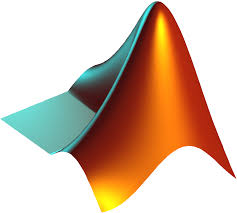Resources

Below are links to various resources for the course.

Documents
Lecture notes

 Lecture 1 Lecture 2 Lecture 3 Lecture 4 Lecture 5 Lecture 6 Lecture 7 Lecture 8 Lecture 9 Lecture 10 Lecture 11 Lecture 11 (Handout) Lecture 12 Lecture 13 Lecture 14 Lecture 15 Lecture 16 Lecture 17 Lecture 18 Lecture 19 Lecture 20 Lecture 21 Lecture 22 Lecture 23 Lecture 24 Lecture 25 Lecture 26 Lecture 27 Lecture 28

Fibonacci sequence

Fibonacci numbers
Golden ratio
Vi Hart - Fibonacci sequence in plants - part I, part II, part III

Giraffes

Giraffes - the underestimated species, NY Times
Artiodactyl brain size evolution, see Figure 3 on page 17 and Appendix B

Wolfram Alpha
Wolfram Alpha is an online answer engine that is very useful for mathematical problems. It can solve linear equations and find eigenvalues, but also differentiate and integrate, plot functions and much more.Wolfram Alpha

Maple
Like Wolfram Alpha, Maple can be used for checking your solutions. At Dartmouth there is licensed software available for students. If you would like to install Maple you can follow the instructions here:Maple

MATLAB

MATLAB, a contraction of "matrix laboratory", is a computing environment and programming language developed by MathWorks. It can be used in a similar fashion as Maple. Dartmouth has a site license for MATLAB. You can download it here:MATLAB

Bjoern Muetzel
Last updated November 12, 2019